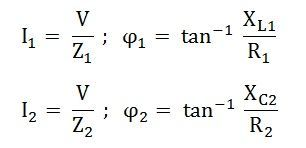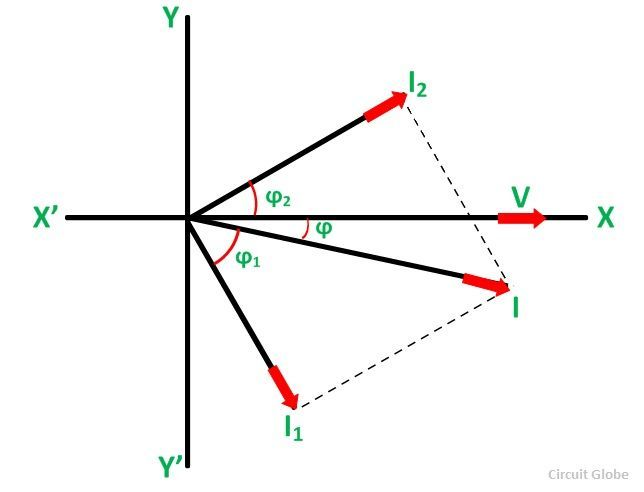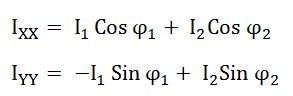# Phasor Method for Solving Parallel Circuits

For solving Parallel Circuits, a number of branches are connected in parallel. Each branch contains a number of components like resistance, inductance and capacitance forming a series circuit. Each branch of the circuit is analysed separately as a series circuit and after that, the effects of each branch are combined together.

For circuit calculations, the magnitude and phase angle of current and voltage is taken into consideration.

The magnitudes and phase angle voltages and currents are taken into consideration while solving the circuit. There are mainly three methods of solving the parallel AC circuits. They are as follows:

• Phasor Method or Vector Method
• Method of Phasor algebra or Symbolic method or J method

The method which yields quick result is applied. In this article, the Phasor Method is explained in detail.

## Steps to Solve Parallel Circuits By Phasor Method

Consider the circuit diagram below to solve the circuit step by step.Step 1 – Draw the circuit diagram as per the given problem. As we are considering the above circuit as an example.

Here, two branches connected in parallel are taken into consideration. One branch contains resistance and inductance in series. The second branch consists of resistance and capacitance in series. The supply voltage is V volts.

Step 2 – Find the impedance of each branch of the circuit separately, i.e.Where, XL1 = 2πfL1Where, XC2 = I/2πfC2

Step 3 – Determine the magnitude of current and phase angle with the voltage in each branch.Here,

ϕ1 is lagging this means it is for inductive load and ϕ2 leading as it is for the capacitive load.

Step 4 – Draw the phasor diagram taking voltage as the reference. Represent the various branch current on it as shown in the phasor diagram below.Step 5 – Now, find the phasor sum of the branch currents by the methods of components.And therefore, current I will beStep 6 – Find the phase angle ϕ between the total current I and the circuit voltage V.Here angle ϕ will be lagging as Iyy is negative

The power factor of the circuit will be Cosϕ orThis is all about the phasor method of solving parallel circuits.